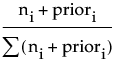The response can be either continuous, or categorical (nominal or ordinal). If Y is categorical, then it is fitting the probabilities estimated for the response levels, minimizing the residual log-likelihood chi-square [2*entropy]. If the response is continuous, then the platform fits means, minimizing the sum of squared errors.
The factors can be either continuous, or categorical (nominal or ordinal). If an X is continuous, then the partition is done according to a splitting “cut” value for X. If X is categorical, then it divides the X categories into two groups of levels and considers all possible groupings into two levels.
-log10(p-value)
where the adjusted p-value is calculated in a complex manner that takes into account the number of different ways splits can occur. This calculation is very fair compared to the unadjusted p-value, which favors Xs with many levels, and the Bonferroni p-value, which favors Xs with small numbers of levels. Details on the method are discussed in a white paper “Monte Carlo Calibration of Distributions of Partition Statistics” found on the JMP website www.jmp.com.
SStest = SSparent - (SSright + SSleft) where SS in a node is just s2(n - 1).
For categorical responses, the G2 (likelihood-ratio chi-square) is shown in the report. This is actually twice the [natural log] entropy or twice the change in the entropy. Entropy is Σ -log(p) for each observation, where p is the probability attributed to the response that occurred.
A candidate G2 that has been chosen is
G2 test = G2 parent - (G2 left + G2 right).
Probi =where the summation is across all response levels; ni is the number of observations at the node for the ith response level; and priori is the prior probability for the ith response level, calculated as
priori = λpi+ (1-λ)Pi
where pi is the priori from the parent node, Pi is the Probi from the parent node, and λ is a weighting factor currently set at 0.9.